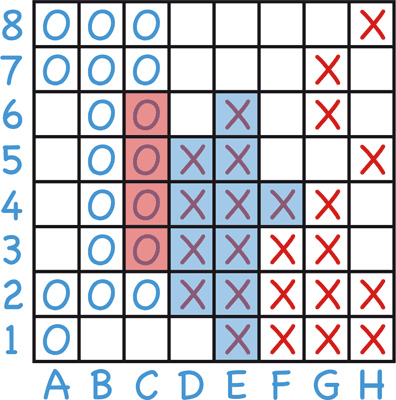## Removing duplicates from sets of three integers

18th May 2020

I've recently encountered an interesting problem while programming some games to add to my Pencil and Paper Games website, papg.com. All the games are written in Lisp, and run on a CL-HTTP server also written in Lisp.

The latest game I've been working on is Virus War:It's an interesting pencil-and-paper game for two players that probably originated in the Soviet Union in the 1970s. It has the unusual feature that each player has three moves in a row. For a full description of the game see Virus War.

The problem is this: given a list of moves, each of which consists of three squares on the board, what's the most efficient way to remove sets of three moves that are equivalent; in other words, consist of the same three moves in a different order?

So, for example, if we have the list of moves:

`((1 2 3) (1 3 4) (3 1 2) (4 1 3) (2 3 4) (3 2 1))`

this can be reduced to:

`((1 2 3) (1 3 4) (2 3 4))`

because all the others are permutations of ones we've already got.

The board has a maximum size of 10 x 10, so each square is identified by a number from 0 to 99. Here's the program I used to generate data to test out some alternative approaches to solving this problem:

```(defparameter *random*
(let (list)
(dotimes (n 10000 list)
(let ((triple (list (random 100) (random 100) (random 100))))
(push triple list)))))```

### Sort triplets with sort and compare with tree-equal

First, the obvious simple-minded solution: use sort to sort each triple into ascending order, and then use tree-equal to compare them:

```(defun test1 (list)
(length
(remove-duplicates
list
:key #'(lambda (x) (sort (copy-seq x) #'<))
:test #'tree-equal)))```

Here's the result:

```User time    =       23.750
Allocation   = 1766959036 bytes```

A delay of 24 seconds is definitely going to make the game unplayably slow!

### Sort triplets more efficiently and compare with tree-equal

Since we've just got three numbers it's inefficient to use sort to sort them. We know that if the numbers are (a b c) the smallest is

`(min a b c)`

the largest is:

`(max a b c)`

and the middle one is :

`(- (+ a b c) (min a b c) (max a b c))`

```(defun sort3 (list)
(let ((max (apply #'max list))
(min (apply #'min list))
(sum (apply #'+ list)))
(list min (- sum min max) max)))```

and:

```(defun test2 (list)
(length
(remove-duplicates
list
:key #'sort3
:test #'tree-equal)))```

This gives the result:

```User time    =       13.755
Allocation   = 1766931512 bytes```

Definitely going in the right direction!

### Convert the three numbers into a product of primes

It would be much more efficient to do an integer comparison, so what we need is a function that takes three integers, and returns an integer that is unique for each possible set of integers, but is unchanged by a permutation of the three integers.

One possible function is to convert each number into the nth prime number, and then multiply them together:

f(a, b, c) = pa x pb x pc

Because multiplication is commutative the result is unaffected by the order of a, b, and c, and because we've chosen prime numbers there will be no other set of a, b, and c that gives the same result.

First we need to create an array of the first 100 prime numbers in *primes*:

```(defun prime-p (n)
(let ((d 2))
(loop
(when (> (* d d) n) (return t))
(when (zerop (mod n d)) (return nil))
(incf d))))

(defparameter *primes*
(let ((a (make-array 100))
(p 2) (i 0))
(loop
(when (prime-p p) (setf (aref a i) p) (incf i) (when (= i 100) (return a)))
(incf p))))```

The 100th prime is 541, and 5413 is smaller than most-positive-fixnum on most Common Lisp implementations, so the integer comparison will be efficient.

Here's the function:

```(defun product-primes (list)
(reduce #'* list :key #'(lambda (y) (aref *primes* y))))```

Check it works:

```> (product-primes '(12 34 56))
1643321

> (product-primes '(56 12 34))
1643321```

Here's the program:

```(defun test4 (list)
(length
(remove-duplicates
list
:key #'product-primes)))```

Timings:

```User time    =        0.018
Allocation   = 417796 bytes```

Now we're getting somewhere!

### Convert the three numbers into single composite

After thinking of the prime number idea I realised there's a simpler function that we can use in the same way, using the max and min approach used earlier:

```(defun composite (list)
(let ((max (apply #'max list))
(min (apply #'min list))
(sum (apply #'+ list)))
(+ (* min 10000) (* (- sum min max) 100) max)))```

This creates a six-digit decimal number containing the three numbers concatenated together in ascending order:

```> (composite '(56 12 34))
123456```

Clearly it has the same properties as product-primes, without needing to build a separate array of prime numbers.

Here's the test program:

```(defun test2 (list)
(length
(remove-duplicates
list
:key #'composite)))```

and the performance is similar:

```User time    =        0.017
Allocation   = 417868 bytes```

### Optimising the final version

We can make composite slightly more efficient as follows:

```(defun composite2 (list)
(let ((max (max (first list) (second list) (third list)))
(min (min (first list) (second list) (third list)))
(sum (+ (first list) (second list) (third list))))
(+ (* min 10000) (* (- sum min max) 100) max)))```

Using this shaves a few milliseconds off the time:

```(defun test6 (list)
(length
(remove-duplicates
list
:key #'composite2)))```

This gives:

```User time    =        0.005
Allocation   = 380816 bytes```

This is nearly 5000 times faster than the simple-minded approach I gave at the start of the article!